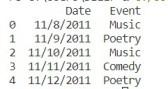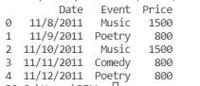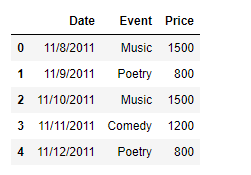GFG App
Open AppBrowser
Continue

# Python | Creating a Pandas dataframe column based on a given condition

While operating on data, there could be instances where we would like to add a column based on some condition. There does not exist any library function to achieve this task directly, so we are going to see the ways in which we can achieve this goal. In this article we will see how to create a Pandas dataframe column based on a given condition in Python.

## Creating a Pandas dataframe column based on a condition

Problem: Given a dataframe containing the data of a cultural event, add a column called ‘Price’ which contains the ticket price for a particular day based on the type of event that will be conducted on that particular day.

## Creating a DataFrame

Here we are creating the dataframe to solve the given problem.

## Python3

 `# importing pandas as pd` `import` `pandas as pd`   `# Creating the dataframe` `df ``=` `pd.DataFrame({``'Date'` `: [``'11/8/2011'``, ``'11/9/2011'``, ``'11/10/2011'``,` `                                        ``'11/11/2011'``, ``'11/12/2011'``],` `                ``'Event'` `: [``'Music'``, ``'Poetry'``, ``'Music'``, ``'Comedy'``, ``'Poetry'``]})`   `# Print the dataframe` `print``(df)`

Output:## Using list comprehension

We can use Python’s list comprehension technique to achieve this task. List comprehension is mostly faster than other methods.
Now we will add a new column called ‘Price’ to the dataframe. For that purpose, we will use list comprehension technique. Set the price to 1500 if the ‘Event’ is ‘Music’ else 800.

## Python3

 `# Add a new column named 'Price'` `df[``'Price'``] ``=` `[``1500` `if` `x ``=``=``'Music'` `else` `800` `for` `x ``in` `df[``'Event'``]]`   `# Print the DataFrame` `print``(df)`

Output :As we can see in the output, we have successfully added a new column to the dataframe based on some condition.

## Using DataFrame.apply() function

We can use DataFrame.apply() function to achieve the goal. There could be instances when we have more than two values, in that case, we can use a dictionary to map new values onto the keys. This does provide a lot of flexibility when we are having a larger number of categories for which we want to assign different values to the newly added column.

Now we will add a new column called ‘Price’ to the dataframe. For that purpose we will use DataFrame.apply() function to achieve the goal. Set the price to 1500 if the ‘Event’ is ‘Music’, 1200 if the ‘Event’ is ‘Comedy’ and 800 if the ‘Event’ is ‘Poetry’.

## Python3

 `# Define a function to map the values` `def` `set_value(row_number, assigned_value):` `    ``return` `assigned_value[row_number]`   `# Create the dictionary` `event_dictionary ``=``{``'Music'` `: ``1500``, ``'Poetry'` `: ``800``, ``'Comedy'` `: ``1200``}`   `# Add a new column named 'Price'` `df[``'Price'``] ``=` `df[``'Event'``].``apply``(set_value, args ``=``(event_dictionary, ))`   `# Print the DataFrame` `print``(df)`

Output :As we can see in the output, we have successfully added a new column to the dataframe based on some condition.

## Using DataFrame.map() function

We can use DataFrame.map() function to achieve the goal. It is a very straight forward method where we use a dictionary to simply map values to the newly added column based on the key.

Now we will add a new column called ‘Price’ to the dataframe. For that purpose we will use DataFrame.map() function to achieve the goal. Set the price to 1500 if the ‘Event’ is ‘Music’, 1200 if the ‘Event’ is ‘Comedy’ and 800 if the ‘Event’ is ‘Poetry’.

## Python3

 `# Create the dictionary` `event_dictionary ``=``{``'Music'` `: ``1500``, ``'Poetry'` `: ``800``, ``'Comedy'` `: ``1200``}`   `# Add a new column named 'Price'` `df[``'Price'``] ``=` `df[``'Event'``].``map``(event_dictionary)`   `# Print the DataFrame` `print``(df)`

Output :## Using numpy.where() function

We can use numpy.where() function to achieve the goal. It is a very straight forward method where we use a where condition to simply map values to the newly added column based on the condition.

Now we will add a new column called ‘Price’ to the dataframe. Set the price to 1500 if the ‘Event’ is ‘Music’, 1500 and rest all the events to 800.

## Python3

 `# np.where(condition, value if condition ` `# is true, value if condition is false)`   `df[``'Price'``] ``=` `np.where(df[``'Event'``] ` `                       ``=``=``'Music'``, ``1500``,``800` `)`   `print``(df)`

Output:My Personal Notes arrow_drop_up